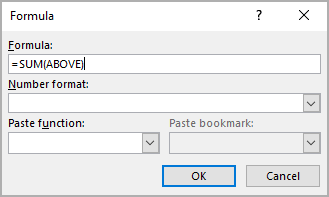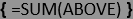# Calculations in the table

Word
You can add, subtract, multiply, and divide numbers in Word table cells. Also, you can calculate averages, percentages, and minimum as well as maximum values.

To add the formula in the Word table, do the following:

1.   Click the table cell in which you want to insert a formula. Word adds Table Design and Layout tabs:2.   On the Table Layout tab, in the Data group, click the Formula button:• If the cell you selected is at the bottom of a column of numbers, Microsoft Word proposes the formula = SUM(ABOVE):• If the cell you selected is at the right end of a row of numbers, Word proposes the formula = SUM(LEFT).

3.   In the Formula dialog box:

• In the Paste function list box, choose a function that you want to add.
• To reference the contents of a table cell, type the cell references in the formula.
The first column in a table is column A; the second column is column B, and so on. The first row is row 1; the second row is row 2, and so on. Thus, the cell in the second column and the third row is cell B3.
For example, to add the numbers in cells B1 and C3, the formula would read = SUM(b1,c3).
• In the Number format list box, choose a format for the numbers.

4.   Click OK.

Note: Word inserts the result of the calculation as a field in the cell you selected. If you change the values in the referenced cells, you can update the calculation by selecting the field and then pressing F9:If you must perform complex calculations in a table, you can create that table in Excel and then link or embed the table in your Word document so that you can update it using Excel.